PPMT Function in Excel• Definition of PPMT Function in Excel
• How to Use PPMT Function in Excel?

Introduction to PPMT Function in Excel

It’s an inbuilt or pre-built integrated Function in excel that is categorized under Financial function.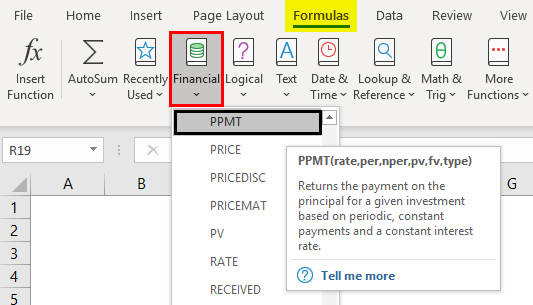Definition of PPMT Function in Excel

PPMT return payment of a principle amount for an investment based on a periodic, constant payment schedule and a fixed interest rate for a given duration or period of time. It indicates how much of the principle amount is being paid in any given pay period.

Syntax of PPMT Function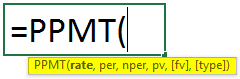Arguments of PPMT Function

• Rate: It’s an interest rate of loan.
• Per (Period): It’s a target or specific payment period, it usually indicates how much principle amount has been repaid.
• Nper (Number of a payment period): Total number of payments to be made for the loan i.e. It is the number of payment periods during the timezone of the loan suppose, if payments are monthly for 3 years, this would be entered 3*12 to calculate 36 payment periods.
• PV (Present value): It is the present value or amount of a loan till now.
• FV (Future value): An optional argument or parameter that indicates the future value of the loan at the end of last or nper payments.
• Type (optional): An optional argument or parameter that indicates when the payments are made, i.e. either at the beginning (1) of a month or at the end (0). If you enter the value 0 or blank value then payments are due at the end of each period. If you enter the value payments are due at the beginning of each period.

Note:

• Rate: It should be either entered as percentage or a decimal number, i.e. if you are making an annual payment at the annual rate of interest of 9%, then it should be entered as 9% OR 0.09 OR monthly payment of the same loan with the same interest rate, then you should enter as 9%/12.
• Per (Period): It should be entered in the range of value 1 to NPER value.
• FV ( Future Value): If you skip or omit this value, [fv] value will be 0 by default.
• Type: If you skip or omit this value, [Type] value will be 0 by default.

How to Use the PPMT Function in Excel?

PPMT Function in Excel is very simple and easy. Let’s understand how to use the PPMT function in Excel with some examples.

You can download this PPMT- Function- Excel -Template here – PPMT- Function- Excel -Template

Example #1 – Calculate the PPMT Value for a 5 Years Duration of Loan

In the below-mentioned example, contains the below-mentioned details of the loan. Here, I need to find out principle amount by using PPMT function.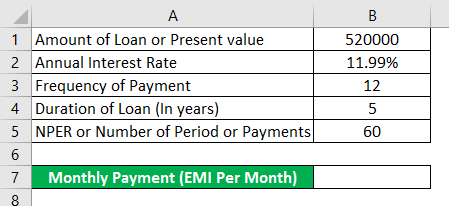• Now, select the “Insert Function” button (fx) option under the formula toolbar, a dialog box will appear, in the search for a function text box, you can enter the keyword “PPMT” and click on go, automatically, function name will appear in “Select a function” box.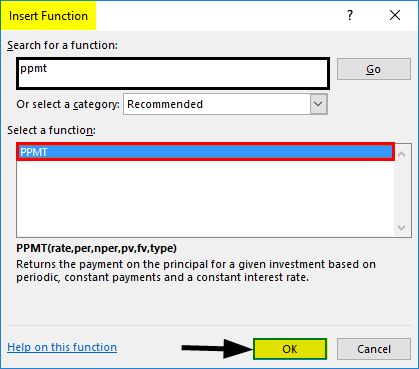Now, you can double click on PPMT function in “Select a function” box. A formula window popup appears where you need to input the formula or syntax argument of PPMT Function. i.e. =PPMT (rate, per, nper, pv, [fv], [type]).

• Rate: It’s an interest rate of a loan, here the annual interest rate is 11.99%, therefore we need to enter it as 11.99%/12.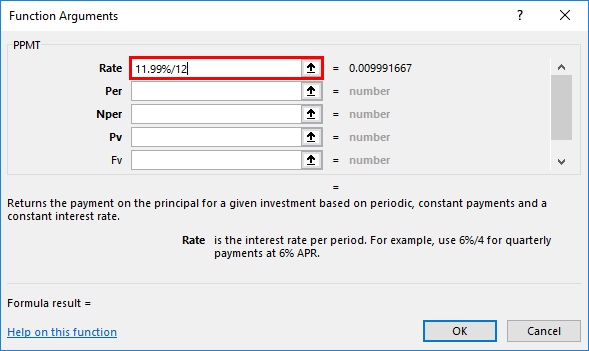• Per: It’s a target or specific payment period i.e. here, the period will be 60 months.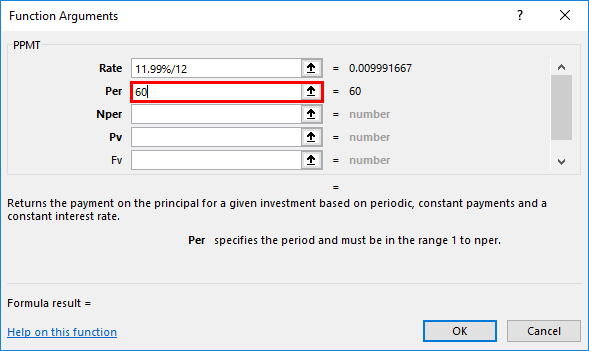• Nper (Number of a payment period): Total number of payments to be made for the loan, Here, the payments are made monthly for 5 years, so it will be 60 months where it should be entered as 12*5.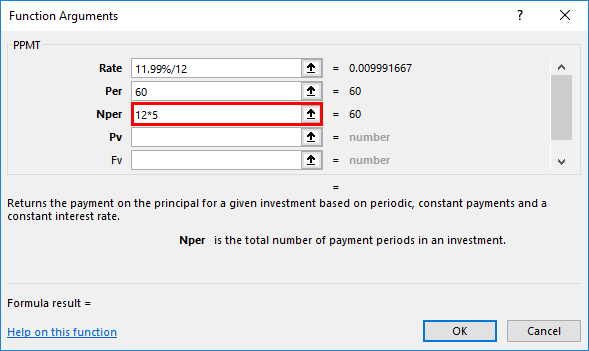• PV (Present value): It is the present value or amount of loan; here you can enter it as a cell reference of the loan amount (C7) or its value 520000.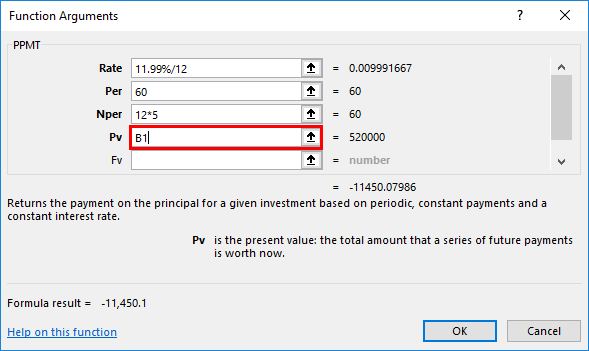• FV (Future value): It is an optional argument, where this parameter can be skipped or omitted, here, you can enter a value as 0 or [fv] value will be 0 by default, we need to assume it as zero balance after the last payment or at the end of NPER payments.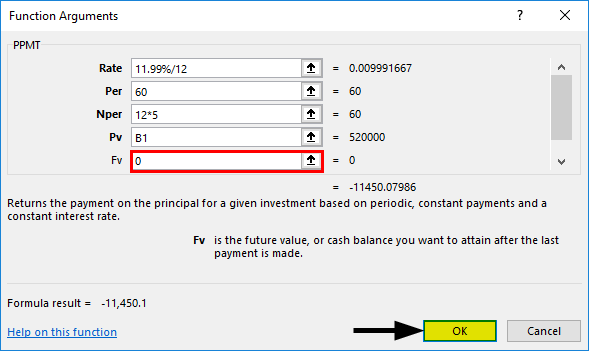• Once after entering all the arguments, we can click on Ok =PPMT (11.99%/12,60,12*5, C7,0) i.e. returns the PPMT or principle amount or value per month. i.e. -11,450 in cell B7.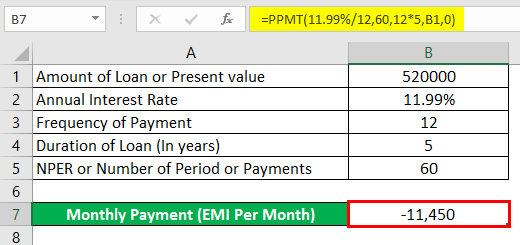Note: It will return the negative value, which indicates outgoing payments, to get a more precise value We have to enter the loan amount i.e. present value as negative. So that it will give a positive value. i.e. =PPMT (11.99%/12,60,12*5, -C7,0)i.e. returns a positive value 11,450.

Example #2 – Calculate the PPMT Value for a 3 Years Duration of Loan

We can calculate the PPMT value for a 3 years duration of loan, just we need to change the PER & NPER values.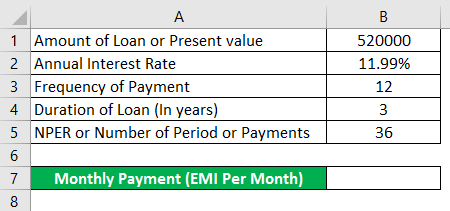Using PPMT Function in cell B7.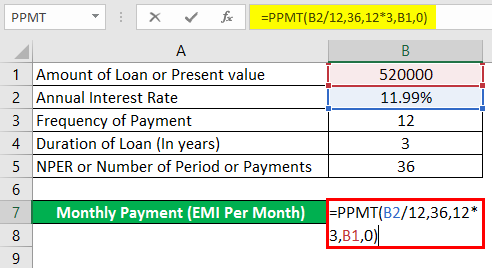After using the PPMT Function in cell B7, answer is shown below.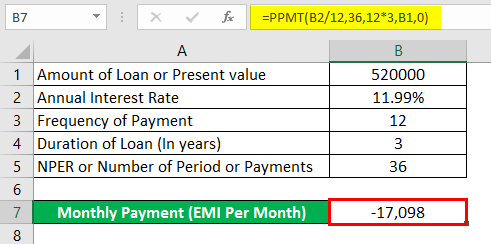i.e. =PPMT (11.99%/12,36,12*3, B1,0) returns the PPMT value -17,098.

Things to Remember

• If you enter any of Values or argument in PPMT as non-numeric, then it will result in “Value error”.
• If the Per (Period) argument in PPMT function is less than 0 or greater than NPER value, then it returns a “#NUM! error”
• You need to enter correct cell reference, If the cell reference is incorrect, then it will return VALUE! error, even if you forget to convert an interest rate or divide the interest rate argument by 12 or 6 0r 4 (based on annual or quarterly payment), it will result in VALUE! error.

Note: It should be always entered in the range of 1 to NPER.

Recommended Articles

This is a guide to PPMT Function in Excel. Here we discuss How to use PPMT function in excel along with practical examples and downloadable excel template. You can also go through our other suggested articles –

1. Excel NORMSINV
2. COUNTA Function in Excel
3. Cell References in Excel
4. Excel IF Function

The post PPMT Function in Excel appeared first on EDUCBA.

This post first appeared on Best Online Training & Video Courses | EduCBA, please read the originial post: here

Subscribe to Best Online Training & Video Courses | Educba

Get updates delivered right to your inbox!

×